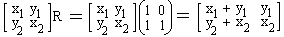# Stern-Brocot Tree

### Binary Encoding

The set of all trees [x, y] forms a two-dimensional vector space with operations of addition and product by a scalar defined naturally as [x1, y1] + [x2, y2] = [x1 + x2, y1 + y2] and s[x, y] = [sx, sy]. Let's denote this space SB. For every tree [x,y], we have also defined its left subtree [x, x + y] and the right subtree [x + y, y]. To formalize the definitions, introduce (linear) operators L and R that act on SB as shown below:

[x, y]L = [x, x + y] and [x, y]R = [x + y,y]

These operators have a matrix representationso that their definition reduces to the product of a vector by a matrix. And there is one more thing. Vector spaces can be added. I am interested here in the direct sum of two copies of SB. Indeed, so far we've been working with sequences of numerators. It's time to apply the theory to sequences of fractions. We shall treat the second copy of SB as a set of trees (or sequences) formed by the denominators. For the convenience sake, I'll write the direct sum of [x1, y1] and [x2, y2] aswhich corresponds to the fact that the "denominator" trees are reflections of the "numerator" trees. (A convenient shorthand is [x1/y2, y1/x2].) Operators L and R are extended to the direct sum SB + SB in a natural wayand similarly for the operator R### Remark

Note that determinants of L and R are both 1: det(L) = det(R) = 1, and recollect that for any two square matrices A and B, det(AB) = det(A)det(B). Therefore, moving from a tree [x1/y2, y1/x2] to either left or right subtree does not change the associated determinant x·x - y·y. For the initial tree [0/1, 1/0], the determinant is -1. This wraps a fact established earlier into the language of linear algebra.After so many definitions we are finally ready for a payoff. In the space SB+SB, the Stern-Brocot tree is represented byCompute, for example, tLRLLThis is the subtree obtained by first selecting the left subtree, then the right subtree of the latter, and, then, the left subtree twice. The tree is defined by two fractions: [1/2, 3/5]! Its stage #0 term is (1 + 3)/(2 + 5) = 4/7. We may think of the fraction 4/7 as being encoded by the sequence LRLL. Whenever encoding starts with R, the fractions are greater than 1. For example, 7/4 is encoded as RLRR. This way, every positive fraction is encoded as a finite sequence of symbols L and R. Can we solve the reverse problem? Given a positive fraction m/n in lowest terms. Find its representation on the Stern-Brocot tree.

The problem is solved by the following algorithm:

### Algorithm 1

while m ≠ n do
if m < n
then (output(L); replace n with n - m)
else (output(R); replace m with m - n)

For example, with m = 4 and n = 7 we successively get

 m 4 4 1 1 1 n 7 3 3 2 1 Output L R L L

To see why the algorithm works, introduce a function f defined for every string S of symbols L and R such that f(S) is exactly the fraction m/n encoded by S. More, accurately, if tS = [m1/n1, m2/n2], then, by definition, f(S) = (m1 + m2)/(n1 + n2).

For m > n, the string encoding for m/n starts with R. Moreover, f(RS) = m/n iff f(S) = (m-n)/n. In other words, f(RS) = f(S) + 1.

### Proof

Assume tS = [m1/n1, m2/n2] and note that, t, when treated as a usual matrix, satisfies t2 = [1/0, 0/1] = I - the identity matrix. This is equivalent to saying that t = t-1, i.e., that t coincides with its own multiplicative inverse. We have S = t[m1/n1, m2/n2] = [n1/m1, n2/m2]. From here RS = [n1/(m1 + n1), n2/(m2 + n2)], and, lastly, tRS = [(m1 + n1)/n1, m2/(m2 + n2)]. f(S) = (m1+n1)/(m2 + n2) is equivalent to f(RS) = (m1+n1+m2 + n2)/(n1 + n2) = (m1 + m2)/(n1 + n2) + 1.

The equivalence follows from an already established fact that every fraction appears in the tree only once and, therefore, uniquely identifies its immediate ancestors.Similarly, if m < n, then the string encoding for m/n starts with L. Moreover, f(LS) = m/n iff f(S) = m/(n - m). This is the same as 1/f(LS) = 1/f(S) + 1. (The two formulas suggest an intimate relationship between the Stern-Brocot tree and Euclid's algorithm.)

The Stern-Brocot tree contains only rational numbers. However, irrational numbers are approximated by rationals. For irrational numbers a, Algorithm 1 is modified (replace m/n with a) to

### Algorithm 2

while (true) do
if a < 1
then (output(L); replace a with a/(1-a))
else (output(R); replace a with a - 1)

In this manner positive irrational numbers are associated with infinite strings of symbols L and R. For example, f(RL0RLR2LRL4RLR6LRL8RLR10...) = e, where powers denote symbol repetition. By taking only finite parts of the string we obtain the following progressively better approximations to e: 1/1, 2/1, 3/1, 5/2, 8/3, 11/4, 19/7, 30/11, 49/18, 68/25, etc.

Algorithm 2 never stops even when a happens to be rational. In the latter case, assuming f(S) = a, Algorithm 2 generates a second representation for a: SRL fully in accordance with what happens in numerical base systems.

## References

1. R.Graham, D.Knuth, O.Patashnik, Concrete Mathematics, 2nd edition, Addison-Wesley, 1994.